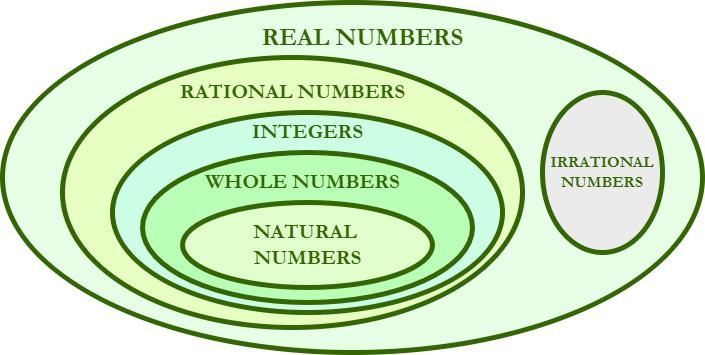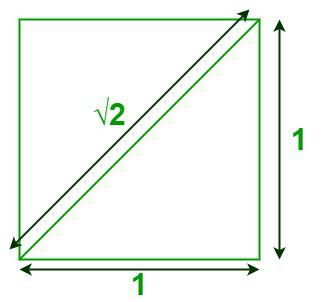Open in App
Not now

# Irrational Numbers

• Last Updated : 15 Sep, 2022

Irrational Numbers are the numbers that can not be expressed in the form of p/q where p and q are integers and q does not equal zero. Irrational numbers cant be represented via a fraction or normal decimals. They are non-recurring and non-repeating decimals. They are part of real numbers but are different from rational numbers. The symbol to represent the Irrational number is Q’.

## What are Irrational Numbers?

Irrational numbers are a special type of number and they can not be expressed as the ratio of two integers. They are a subset of Real Numbers and can be expressed on the number line. And, the decimal expansion of an irrational number is neither terminating nor repeating.

Irrational numbers are real numbers that cannot be expressed as p/q where p and q are integers and q does not equal zero. They can not be expressed as repeating and terminating Decimals.

## How to Identify an Irrational Number?

We know that irrational numbers are real numbers and they cannot be expressed in the form of p/q, where p and q are integers and q ≠ 0. For example, √ 5 and √ 3, etc. are irrational numbers. On the other hand, the numbers which can be represented in the form of p/q, such that, p and q are integers and q ≠ 0, are rational numbers.

## Irrational Numbers Symbol

√2, √3, π, e are some examples of irrational numbers.

• √2 = 1.41421356237309504880…
• Pi “π”= The value of π is 3.14159265358979323846264338327950… It is a really famous irrational number. People have calculated its value up to quadrillion decimal but still haven’t found any pattern yet.
• Euler’s number  “e” = Euler number is also very popular in mathematics. In this case, also, people tried calculating it up to a lot of decimals but still, no pattern was found. the value of e = 2.7182818284590452353602874713527 (and more …).
• Golden ratio  “ϕ” = This is an irrational number and its application is found in many fields like computer science, design, art, and architecture.

## Are Irrational Numbers Real Numbers?

Irrational numbers come under real numbers, i.e. all irrational numbers are real. But irrational numbers are different from rational numbers as they can’t be written in the form of fractions. The differences between rational and irrational numbers can be learned here. Although, irrational numbers can be expressed in the form of non-terminating and non-recurring fractions. For example,  √2, √3, and π are all irrational numbers and can’t be written as fractions. The image below explains the relationship between Irrational numbers and Real Numbers.## How to say √2 is an Irrational number?Suppose it is a Rational Number

Since it is Rational, It can be represented in the most simplified form P/Q where P/Q are Integers (Q≠ 0) and P/Q can not be further simplified,

Which means that the fraction P/Q is irreducible.

P/Q = √2

Squaring both Sides:

(P/Q)2 = 2

P2/Q2 = 2

P2 = 2 Q2

Here, It is clear that P2 is divisible by 2. Therefore, P2 is an Even Number.

Since, P2 is Even, P has to be an Even number too.

Therefore, P can be written as 2A as it is divisible by 2.

By putting the value P = 2A, we will get:

(2A)2 = 2Q2

4A2 = 2Q2

2A2 = Q2

Here, It is observed that Q2 is also an Even number as it is divisible by 2.

Since Q2 is Even, Q has to be Even too. Therefore, Both P and Q turned out to be Even numbers, which means they can be Simplified further.

It is a Contradiction as it was already defined to be a fraction in its simplest form.

Therefore, √2 cannot be Rational, It is an Irrational number.

## Properties of Irrational Numbers

Various properties of irrational numbers are discussed below:

### Addition of an Irrational and Rational number

The addition of an irrational and a rational number always gives an irrational number.

For example, it’s known that √2 = 1.41421356237309504880… Now √2 + 1 = 2.41421356237309504880…. This is still irrational.

### Multiplication of an irrational number with a non-zero rational number

Multiplication of any irrational number with any nonzero rational number results in an irrational number.

Proof:

Let x be an irrational number and y be a non-zero rational number.

We want to know if z = xy is irrational or rational ?

This will be proved by contradiction. Let’s assume z is rational number.

If z is rational, then x = z/y where both z and y are rational number. This makes x as rational number. This is a contradiction, which means that the previous assumption was wrong. So, z will always be irrational number.

### Product of two Irrational Numbers

When multiplying a rational number, it is not necessary that the resulting number is always irrational.

• π × π = π2 is irrational.
• But √2 × √2 = 2 is rational.

So Product of two Irrational Numbers can result in Rational or Irrational Number accordingly

### Sum of Two Irrational Numbers

The answer to this is also similar to the above property. The Sum of two irrational numbers is sometimes rational sometimes irrational.

• 3√2 + 4√3 is irrational.
• (3√2 + 6) + (- 3√2) = 6, this is rational.

Fun Facts

Apparently Hippasus (one of Pythagoras’ students) discovered irrational numbers when trying to write the square root of 2 as a fraction (using geometry, it is thought). Instead, he proved the square root of 2 could not be written as a fraction, so it is irrational.But followers of Pythagoras could not accept the existence of irrational numbers, and it is said that Hippasus was drowned at sea as a punishment from the gods!

## Mystery of Pi

Let’s take a circle, measure its circumference, and divide it by its diameter. It will always be a constant if measured accurately.

This constant ratio is denoted by the greek symbol π (read as pi). That is,

Circumference/diameter = π

It is an important universal constant, it occurs in lots of places in our universe and in our daily lives. It was not created by humans, it was discovered. We discovered one of the places where pi occurred, it was geometry.

So, what is the value of Pi?

π = 3.14159265358979323846264338327950…

It is not infinite, it is an irrational number.

Note: We often take 22/7 as the value of Pi, but it is an approximation.

Now one might think, how is pi irrational? One can measure the circumference, One can measure the diameter, and then take their ratio. So it must be rational. Actually, no one can encounter such a case, if the diameter is measured and it is rational. Then circumference must be irrational and vice versa. So either diameter or circumference. One of them will always be irrational. Usually, the measuring instruments are not precise enough. If there existed a perfect measuring scale, it would tell that at least one of the numbers in the fraction is irrational.

## Solved Examples on Irrational Numbers

Example 1: Find Rational Numbers or Irrational Numbers among the following.

2, 3, √3, √2, 1.33333…, 1.1121231234…

Solution:

Rational Numbers: 2, 3, 1.3333…. are rational numbers

Irrational Numbers: √3, √2, 1.1121231234… are irrational numbers

Example 2: Find the sum of the following irrational numbers.

a) √2, √2  b) √2, √3

Solution:

a) √2 + √2 = 2√ 2 (they are added as two like variables)

b) √2 + √3 = √2 + √3 (they can’t be added as unlike variables)

Example 3: Find the product of the following rational numbers.

a) √2, √2  b) √2, √3

Solution:

a) √2 × √2 = 2

b) √2 × √3 = √6

## FAQs on Irrational Numbers

Question 1: Do these Numbers come under the category of Irrational numbers: 5, 3.45, 4.444444…, √9.

These numbers mentioned above are not Irrational number.

• 5 is a Whole number and therefore, is Rational
• 3.45 is a number with Terminating Decimal and therefore, it is Rational too.
• 4.444444… is a Number with Repeating Decimal Expansion, It is Rational.
• √9 is 3 i.e; the square root of 9 is 3 and 3 is a Whole number. Therefore, √9 is Rational.

Question 2: “Every real number is an irrational number”. True or False?

False, All numbers are real numbers and all non-terminating real numbers are irrational number. For example 2, 3, 4, etc. are some example of real numbers and these are not irrational.

Question 3: Determine whether the following numbers are Rational or Irrational.

√3, 74, 8.432432432…, 3.14159265358979…, √11, 55/5.

74, 8.432432432…, and 55/5(11) are Rational numbers as either they are Integers or their decimal expansions are terminating, repeating.

√3, 3.14159265358979…, and √11 are Irrational numbers as their decimal Expansions are Non-terminating, Non-repeating.

Question 4: Why Integers are not Irrational numbers?

Integers (Either Positive, Negative or Zero) are not Irrational but Rational numbers since they can be represented in the simplest fraction form P/Q (where Q ≠ 0).

Question 5: Write some commonly used irrational numbers.